Shooting method

A method for solving initial and boundary value problems for ordinary differential equations. It consists of introducing control variables (parameters) and subsequently determining them from the system of equations, where this choice of parameters has a decisive influence on the acceleration of the solution of the system.

Suppose, for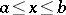, one is given the differential equation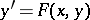(1)

with boundary condition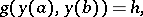(2)

where the vector functionofis to be determined, the vector functions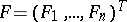and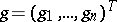are known, and the numerical vector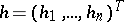is given.

Suppose that the Cauchy problem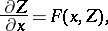(3)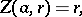(4)

whereand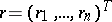, has a unique solution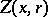, defined for,. Upon substituting in (2) for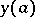the given value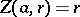and forthe calculated value, one obtains the equation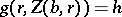(5)

with respect to the parameter.

The algorithm of the shooting method is as follows. First one finds a solutionof (5), and subsequently the required solution of the boundary value problem (1)–(2) as the solution of the Cauchy problemThis problem can be solved using numerical methods. In order to solve (5) it is usually necessary to choose some iteration method.

If some of the components ofdepend onalone and the others on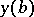alone, it is useful to make another choice of parameters (cf. , as well as Non-linear boundary value problem, numerical methods). There are other versions of the shooting method (cf. ). The shooting method is also used in solving grid boundary value problems.